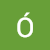Flashcards by Jonathan Griffin, updated 7 months ago More LessCreated by Jonathan Griffin over 1 year ago100

### Description## Resource summary

 Question Answer Where is the zero force member in a 3 member joint? Analogy? If no external load, the member that is not co linear is zero force. Its the I in the T. Given a laterally loaded frame of Width = 4m and H = 3m, which method of Approx. Analysis would be used, why and what assumptions? As ratio is <2 it is low elevation. Shear is key in short beams so use Portal Method. Assume; - Hinge at centre of beams and columns - Shear is double on internal columns First step for Portal Method when initially analysing a laterally loaded frames 'floor' section? So, for the next floor? Sum Fx to find initial Shear Reaction. Repeat on next floor. (Portal) When analysing lower floors, what are the Shear forces resisting? The sum of P's on all floors above. Which method? First Step? General Procedure? Cantilever+1 (binary/octet-stream) Cantilever Method 1st: Find the Centroid of the frame. 2nd: Take M about centroid, find verticals in terms of 1st Column, Solve for Ay, then statics. Cantilever+2 (binary/octet-stream) Cantilever Method Assumptions? Hinges in Beams/Columns Force is proportional to distance from Centroid How find Centroid? (Formula) where x is distance from x=0 Centroid (binary/octet-stream) Key difference in 1st assumption between Portal and Cantilever Methods? Portal assumes to find Fx Cantilever assumes to find Fy

### Similar

I'm the king of the castle
Plant and animal cells
Carmichael et al (1932) - Edexcel GCSE Psychology
A View from the Bridge Quotes
GCSE Biology B1 (OCR)
Astronomy Practice Quiz
Simple Present Tense (Test)
The Tempest
AWS – Solution Architect Associate Level Certification - Mock Test
The sign of four themes
Macbeth Key Quotes